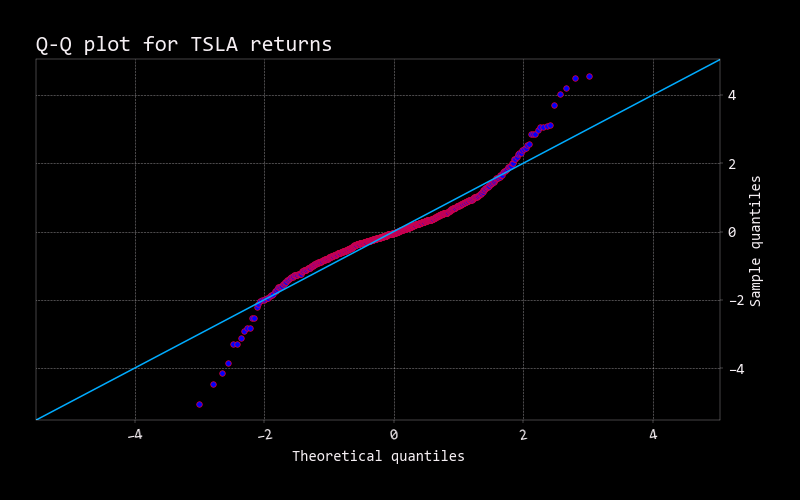# Qqplot

``````usage: qqplot [-h]
``````

Display Q-Q plot vs normal quantiles. See the Wiki page on this topic here: https://en.wikipedia.org/wiki/Q–Q_plot

A Q–Q plot is used to compare the shapes of distributions, providing a graphical view of how properties such as location, scale, and skewness are similar or different in the two distributions. Q–Q plots can be used to compare collections of data, or theoretical distributions. The use of Q–Q plots to compare two samples of data can be viewed as a non-parametric approach to comparing their underlying distributions. A Q–Q plot is generally a more powerful approach to do this than the common technique of comparing histograms of the two samples, but requires more skill to interpret. Q–Q plots are commonly used to compare a data set to a theoretical model.

The points plotted in a Q–Q plot are always non-decreasing when viewed from left to right. If the two distributions being compared are identical, the Q–Q plot follows the 45° line y = x. If the two distributions agree after linearly transforming the values in one of the distributions, then the Q–Q plot follows some line, but not necessarily the line y = x. If the general trend of the Q–Q plot is flatter than the line y = x, the distribution plotted on the horizontal axis is more dispersed than the distribution plotted on the vertical axis. Conversely, if the general trend of the Q–Q plot is steeper than the line y = x, the distribution plotted on the vertical axis is more dispersed than the distribution plotted on the horizontal axis. Q–Q plots are often arced, or “S” shaped, indicating that one of the distributions is more skewed than the other, or that one of the distributions has heavier tails than the other.

Although a Q–Q plot is based on quantiles, in a standard Q–Q plot it is not possible to determine which point in the Q–Q plot determines a given quantile. For example, it is not possible to determine the median of either of the two distributions being compared by inspecting the Q–Q plot. Some Q–Q plots indicate the deciles to make determinations such as this possible.

The intercept and slope of a linear regression between the quantiles gives a measure of the relative location and relative scale of the samples. If the median of the distribution plotted on the horizontal axis is 0, the intercept of a regression line is a measure of location, and the slope is a measure of scale. The distance between medians is another measure of relative location reflected in a Q–Q plot. The “probability plot correlation coefficient” (PPCC plot) is the correlation coefficient between the paired sample quantiles. The closer the correlation coefficient is to one, the closer the distributions are to being shifted, scaled versions of each other. For distributions with a single shape parameter, the probability plot correlation coefficient plot provides a method for estimating the shape parameter – one simply computes the correlation coefficient for different values of the shape parameter, and uses the one with the best fit, just as if one were comparing distributions of different types.

Another common use of Q–Q plots is to compare the distribution of a sample to a theoretical distribution, such as the standard normal distribution N(0,1), as in a normal probability plot. As in the case when comparing two samples of data, one orders the data (formally, computes the order statistics), then plots them against certain quantiles of the theoretical distribution.

``````optional arguments:
-h, --help  show this help message (default: False)
``````